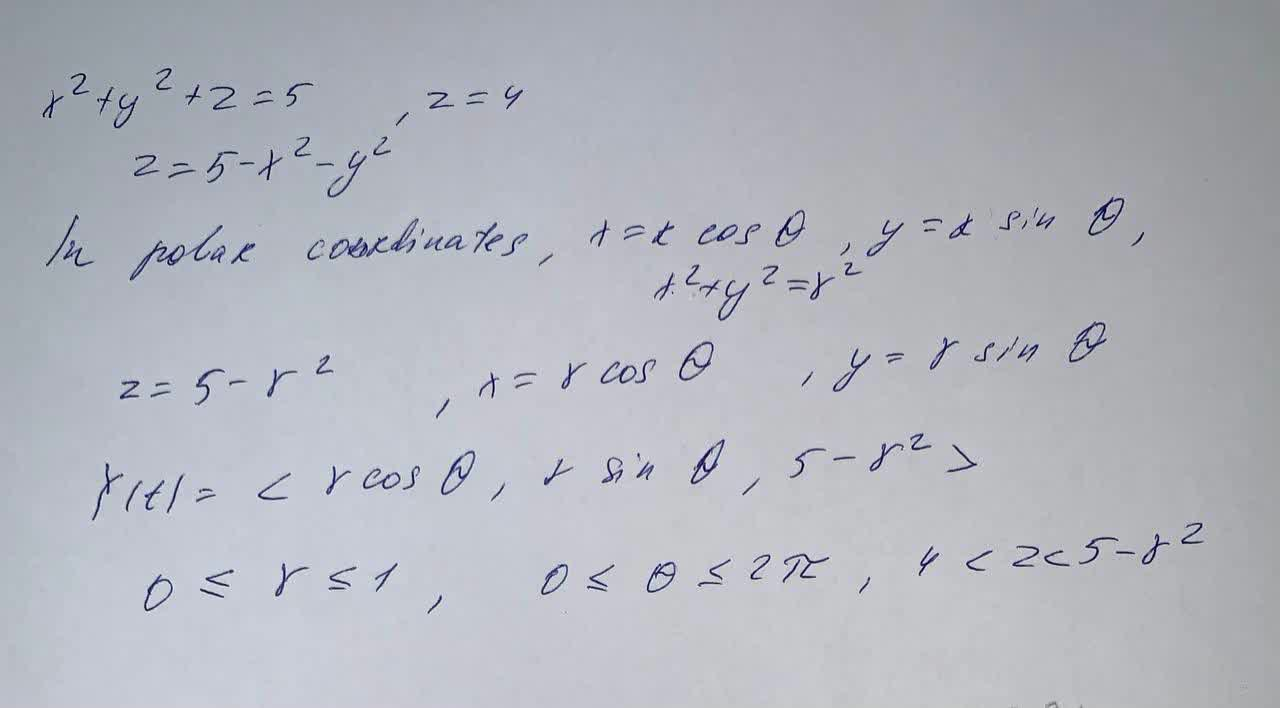# Find parametric equations for x, y, and z in terms of the polar coordinates r and \theta todetermine the points on the portion of the paraboloid x + y + z = 5 that is on or above the planez=4coexpennan 2021-09-14 Answered

Find parametric equations for x, y, and z in terms of the polar coordinates r and $$\displaystyle\theta$$ to determine the points on the portion of the paraboloid $$x + y + z = 5$$ that is on or above the plane $$z=4$$

• Questions are typically answered in as fast as 30 minutes

### Plainmath recommends

• Get a detailed answer even on the hardest topics.
• Ask an expert for a step-by-step guidance to learn to do it yourself.hajavaF

Now we have